# Join, Left, Mid, Right, Find, Substitute functions in Excel

##### How to use Join, Find, Left, Right, Mid, Substitute functions in Excel?

In the previous post, we learnt about Date & Time functions in Excel. In this post, we shall learn about Text manipulation in Excel.

### Join strings in Excel

To join two strings in Excel, we an use '&' operator.

In below example, D3 has formula '=B3 & " " & C3' that concatenates B3 and C3 cell values.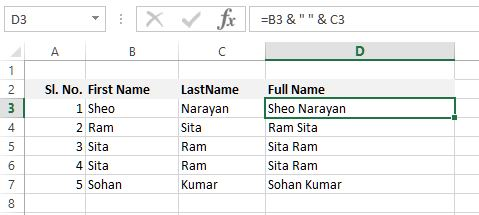To insert more than 1 space use multiple blank space between double quotes like "    ". To know how to copy the formula to other D column cells, read this.

### LEFT function

LEFT function in Excel is used to return the specificed number of characters from start of a string. See E3 cell, we have written '=LEFT(D3, 5)' that returns 'Sheo ' (with space).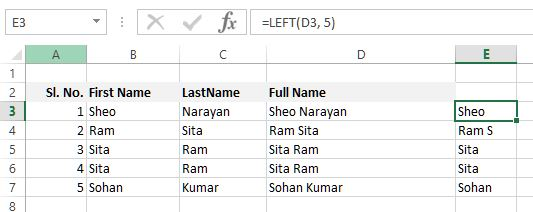The same formula has been copied to other E cells to bring above result.

### RIGHT function

RIGHT function in Excel is used to return specified number of characters from the end of string. E3 cell in below example has '=RIGHT(D3, 5)' formula that returns 5 characters from the end of string of D3 cell.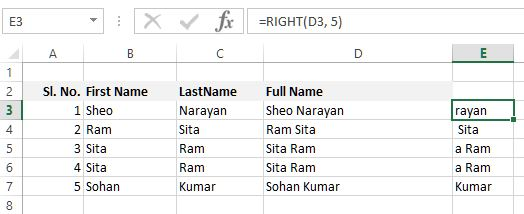The same formula has been copied to other E cells.

### MID function

MID function in Excel is used to return the characters from the middle of string by giving starting position and length of characters to return.

E3 cell in below example has '=MID(D3, 3, 5)' that returns string starting from 3rd position to next 5 characters.Copy, the same formula in E cells to get above result.

### FIND function

FIND function in Excel is used to return the starting position of the 1st occurence of a substring within another string. FIND is a case-sensitive function ie. "an" is difference than "AN".

E3 cell in below example contains '=FIND("an", D3)' formula that finds "an" at the 11th position, so the result is 11.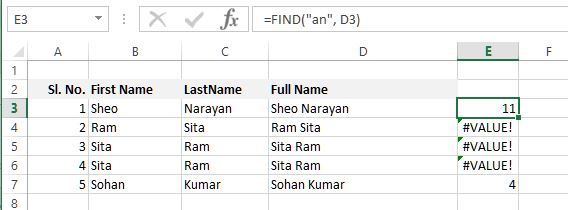If FIND function can't find the specified substring, it throws "#VALUE!" error as shown above.

### SUBSTITUTE function

SUBSTITUTE function in Excel is used to replace a part of text with new text in a string. E3 cell in below example contains '=SUBSTITUTE(D3, " ", "|")' formula that replaces " " (blank space) with "|" (pipe sign).

SUBSTITUTE function is also case-sensitive.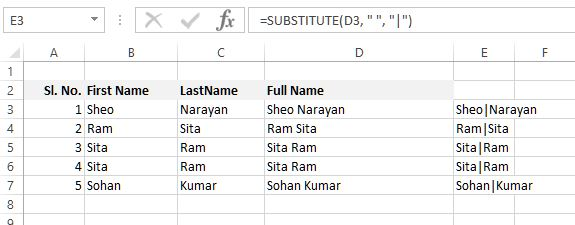The same formula has been copied to other E cells.

Thanks for reading. If you liked it share, please share with friends and colleagues.

Views: 6178 | Post Order: 23